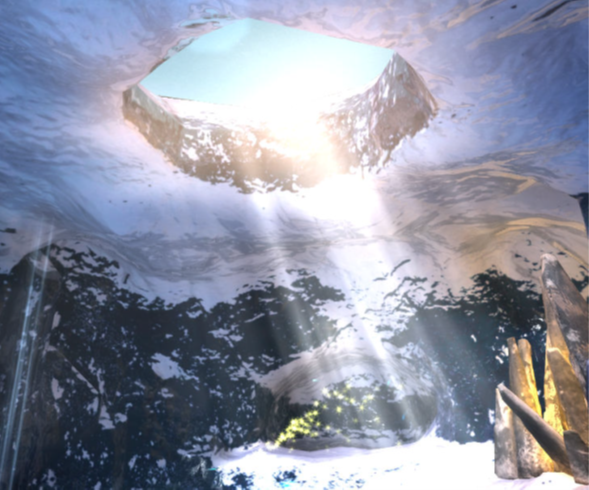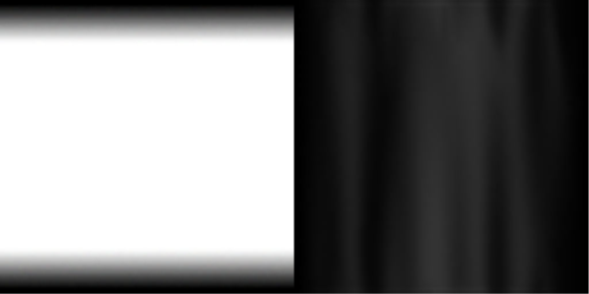## Light shafts

This section of the guide shows you how to implement light shafts into your Unity programs. Light shafts can be used to simulate the following effects:

• Crepuscular rays
• Atmospheric scattering

Implementing the preceding list of effects can add depth and realism to a scene.

In the Ice Cave demo, the light shaft simulates the scattering of the sun rays that are coming into the cave from the opening at the top of the cave.

The following image shows the light shafts in the Ice Cave demo:The light shaft is based on a truncated cone. The cone follows the orientation of the light rays in a manner that ensures that the upper section of the cone is always fixed.

The following diagram shows the geometry of the cone where:

• A shows that the basic geometry of the light shaft is a cylinder with two cross-sections at the top and bottom.
• B shows that the lower cross-section is expanded to achieve a truncated cone geometry based on the angle θ that you define.
• C shows that the upper cross-section is fixed, and that the lower cross-section is shifted horizontally according to the direction of the sun rays.A script can use the position of the sun to calculate the following values:

• The magnitude of the lower cross-section cone expansion based on the value of the angle θ that is provided as input.
• The direction and magnitude of the cross-section shift.

The vertex shader applies a transformation based on this data. The transformation is applied to the original vertices of the cylindrical geometry, in the local coordinates of the light shaft.

When rendering the light shaft, avoid rendering any hard edges that reveal its geometry. You can avoid rendering these edges by using a texture mask that smoothly fades out the top and bottom.

The following image shows the light shaft textures:#### Fading out the cone edges

The following steps show you how to fade out the cone edges. This means that you fade out the light shaft intensity in the plane parallel to the cross section. The intensity of fade out depends on the relative camera-to-vertex orientation.

1. Project the camera position on the cross section, in the vertex shader.
2. Build a new vector from the center of the cross section to the projection and normalize it.
3. Calculate the dot product of this vector with the vertex normal, then raise the result to a power exponent.
4. Pass the result to the fragment shader as a varying. The result is used to modulate the intensity of the light shaft.

The vertex shader performs these calculations in the Local Coordinate System (LCS).

The following code shows the vertex shader:

```// Project camera position onto cross section
float3 axisY = float3(0, 1, 0);
float dotWithYAxis = dot(camPosInLCS, axisY);
float3 projOnCrossSection = camPosInLCS - (axisY * dotWithYAxis);
projOnCrossSection = normalize(projOnCrossSection);
// Dot product to fade the geometry at the edge of the cross section
float dotProd = abs(dot(projOnCrossSection, input.normal));
output.overallIntensity = pow(dotProd, _FadingEdgePower) * _CurrLightShaftIntensity;
```

The coefficient `_FadingEdgePower` enables you to fine-tune the fading of the light shaft edges.

The script passes the coefficient `_CurrLightShaftIntensity`. Passing the coefficient makes the light shaft fade out when the camera gets close to it.

The following code shows a final touch that is added to the light shaft by slowly scrolling down the texture from a script:

```void Update()
{
float localOffset = (Time.time * speed) + offset;
localOffset = localOffset % 1.0f;
GetComponent().material.SetTextureOffset("_MainTex", new Vector2(0, localOffset));
}
The fragment shader fetches the beam and mask textures, and then combines them with the intensity factor:
float4 frag(vertexOutput input) : COLOR
{
float4 textureColor = tex2D(_MainTex, input.tex.xy);
textureColor.rgb = clamp(textureColor.a, 0, 1);
return textureColor;
}
```

The light shaft geometry is rendered in the transparent queue after all the opaque geometry.

The light shaft uses additive blending to combine the fragment color with the corresponding pixel in the frame buffer.

The shader also disables culling and writing to depth buffer, so that it does not occlude other objects.

The settings for the pass are the following:

```Blend One One
Cull Off
ZWrite Off
```